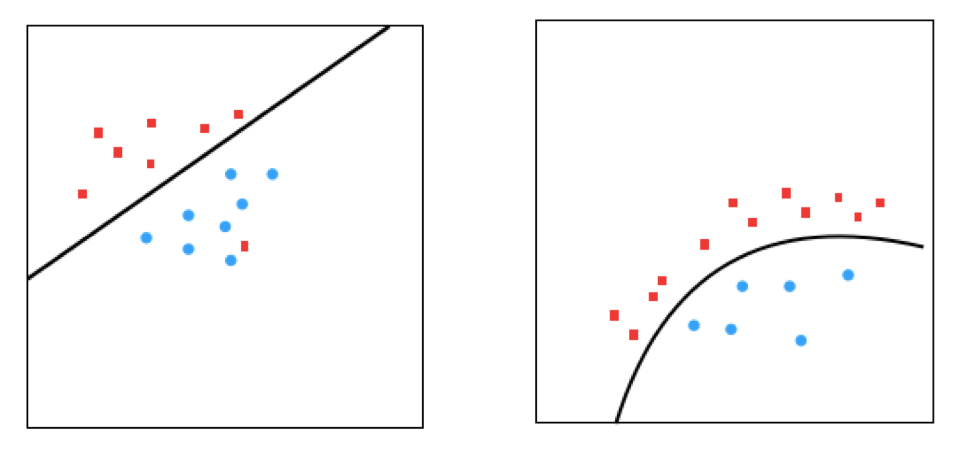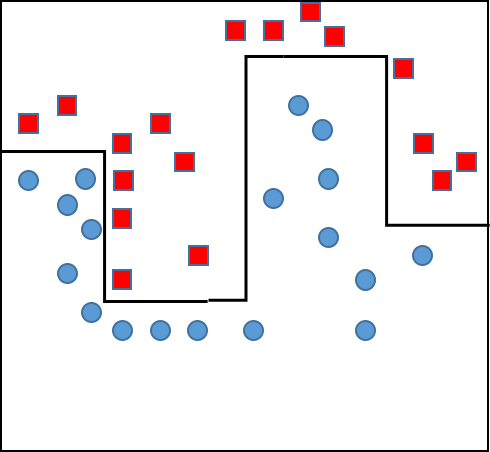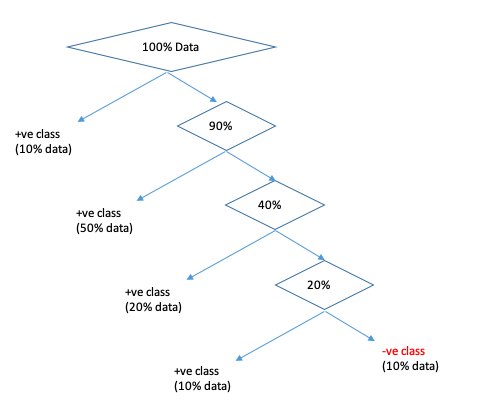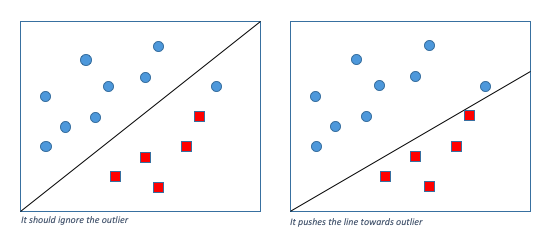{{announcement.body}}
{{announcement.title}}

# Logistic Regression vs. Decision Tree

DZone 's Guide to

# Logistic Regression vs. Decision Tree

### Find the best method for classification based on your data.

· Big Data Zone ·
Free Resource

Comment (1)

Save
{{ articles.views | formatCount}} Views

## When to Use Each Algorithm

Logistics Regression (LR) and Decision Tree (DT) both solve the Classification Problem, and both can be interpreted easily; however, both have pros and cons. Based on the nature of your data choose the appropriate algorithm.

Of course, at the initial level, we apply both algorithms. Then, we choose which model gives the best result. But have you ever thought of why a particular model is performing best on your data?

Let's look at some aspects of data.

### Is Your Data Linearly Separable?

Logistic Regression assumes that the data is linearly (or curvy linearly) separable in space.Decision Trees are non-linear classifiers; they do not require data to be linearly separable.When you are sure that your data set divides into two separable parts, then use a Logistic Regression. If you're not sure, then go with a Decision Tree. A Decision Tree will take care of both.

### Check Data Types

Categorical data works well with Decision Trees, while continuous data work well with Logistic Regression.

If your data is categorical, then Logistic Regression cannot handle pure categorical data (string format). Rather, you need to convert it into numerical data.

1. Enumeration: If we enumerate the labels eg. Mumbai — 1, Delhi — 2, Bangalore — 3, Chennai — 4, then the algorithm will think that Chennai (2) is twice large as Mumbai (1).

2. One Hot Encoding: For the above problem, use One Hot Encoding; however, this could result in a Dimension problem. Therefore, if you have lots of categorical data, go with a Decision Tree.

### Is Your Data Highly Skewed?

Decision Trees handle skewed classes nicely if we let it grow fully.

Eg. 99% data is +ve and 1% data is –veSo, if you find bias in a dataset, then let the Decision Tree grow fully. Don’t cut off or prune branches. Instead, identify max depth according to the skew.

Logistic Regression does not handle skewed classes well. So, in this case, either increase the weight to the minority class or balance the class.

### Does Your Data Contain Outliers?

Logistic regression will push the decision boundary towards the outlier.While a Decision Tree, at the initial stage, won't be affected by an outlier, since an impure leaf will contain nine +ve and one –ve outlier. The label for the leaf will be +ve, since the majority are positive.

However, if we let the Decision Tree grow fully, the signal will mote to one side, while the outlier will be moved to the other — there will be one leaf for each outlier.

### Does Your Data Contain Many Missing Values?

Logistic Regression does not handle missing values; we need to impute those values by mean, mode, and median.

If there are many missing values, then imputing those may not be a good idea, since we are changing the distribution of data by imputing mean everywhere.

Decision Trees works with missing values.

## Cheatsheet.

 Linearly non - separable? Decision Tree Categorical Data type Decision Tree Continuous Data type Logistic Regression Skewed Decision Tree, or give high weight to minority class in Logistic Regression Outlier Decision Tree, or remove outlier for Logistic Regression Lots of Missing values Decision Tree
Topics:
logistic regression ,decision trees ,big data ,skew ,tutorial

Comment (1)

Save
{{ articles.views | formatCount}} Views

Opinions expressed by DZone contributors are their own.